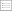Print this page

DISCUSSION PAPER PI-0815

Optimal asset allocation strategy for defined-contribution pension plans with power
utility

Qing-Ping Ma

Optimal asset allocation strategies of defined-contribution pension plans for members
whose terminal utility is a power function of wealth-to-wage ratio is investigated in
this paper. The portfolio problem is to maximize the expected terminal utility in the
presence of three risk sources, interest risk, asset risk and wage risk. A closed form
solution is found for the asset allocation problem and the optimal portfolio
composition is horizon independent when there is no non-hedgeable wage risk or
there is no further contribution from wage incomes. When future contributions from
wage income are hedged by short-selling a wage replicating portfolio, the optimal
composition of financial wealth on hand (i.e. pension portfolio wealth + short-sold
wage replicating portfolio) is horizon-dependent. The optimal asset allocation strategy
is equivalent to invest in two mutual funds, one of which is to hedge wage risk and the
other a speculative fund to satisfy the risk appetite of the plan member.

Keywords : Defined-contribution pension plan; Wage risk; Optimal asset allocation;
Power utility; Hamilton-Jacobi-Bellman equation